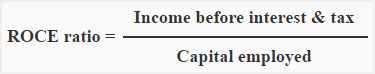# Return on capital employed ratio

Return on capital employed ratio is computed by dividing the net income before interest and tax by capital employed. It measures the success of a business in generating satisfactory profit on capital invested. The ratio is expressed in percentage.

## Formula:The basic components of the formula of return on capital employed ratio are net income before interest and tax and capital employed.

Net income before interest and tax (Operating income):
Net income before the deduction of interest and tax expenses is frequently referred to as operating income. Here, interest means the interest on long term loans. If company pays interest expenses on short-term borrowings, that is deducted to arrive at operating income.

Capital employed:
Capital employed is calculated in a number of ways. Some popular methods are given below:

1. Total of fixed and current assets.
2. Total of fixed assets only.
3. Fixed assets plus working capital.
4. Total of long term funds. Long term funds include capital, Reserve and surplus etc.

In managerial accounting, the last method is usually used to calculate capital employed.

A D V E R T I S E M E N T

## Example:

Fixed assets: \$800,000

Current assets: \$300,000

Long-term investment: \$200,000

Share capital: \$600,000

8% bonds: \$400,000

Reserves: \$200,000

Accounts payable: \$100,000

Net income before interest and tax: \$80,000

From the above information, we can compute the return on capital employed ratio as follows:

= (80,000 / 1,000,000*)

= 0.08 or 8%

*Computation of capital employed: Fixed assets + Current assets – Current liabilities

= \$800,000 + \$300,000 – \$100,000

= \$1,000,000

## Significance and Interpretation:

Return on capital employed ratio measures the efficiency with which the investment made by shareholders and creditors is used in the business. Managers use this ratio for various financial decisions. It is a ratio of overall profitability and a higher ratio is, therefor, better.

To see whether the business has improved its profitability or not, the ratio can be calculated for a number of years.

A D V E R T I S E M E N T
One Comment on Return on capital employed ratio
1.Rue

Thanks